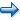# Creating Trials

Now it's time to define the trials for the covert attention task. The trial element specifies which stimuli should be presented, when they are presented, and how the subject should respond. The trial element brings all of the pieces together into a task.

First, we'll define a set of practice trials for the spatial judgment task. The practice trials present our instructions text stimuli that remind the subject how to perform the task.

```<trial topleftpractice>
/ stimulustimes = [0=fixation; 500=leftarrow; 700=toplefttarget, bottomleft, topright, bottomright; 1000=instructleft, instructright]
/ correctresponse = ("a")
/ validresponse = ("s", "a")
/ beginresponsetime = 700
</trial>
```

The "stimulustime" command defines the sequence of stimuli to be presented. The trials presents the fixation point at the beginning of the trial, followed 500 milliseconds later by the left arrow picture. After another 200 milliseconds, the trial presents the target stimulus in the topleft corner and distractor stimuli in other corners. Finally, 300 milliseconds later, the instruction text is presented.

On this trial, the subject can respond by pressing either the "s" or "a" key as defined by the validresponse command. A response of "s" is considered correct as defined by the correctresponse command.

Finally, the beginresponsetime command indicates that Inquisit should start measuring the subject's response 700 milliseconds into the stimulus sequence. This corresponds exactly to the time at which the target and distractor stimuli are presented. Response latencies will be reported relative to this point in time. Responses given before this point are ignored.

The remaining practice trials are similar, except that they present the target in different screen locations and therefore. Depending on whether the target is presented on the left or right side of the screen, either "s" or "a" is defined as the correct response.

```<trial bottomleftpractice>
/ stimulustimes = [0=fixation; 500=leftarrow; 700=topleft, bottomlefttarget, topright, bottomright; 1000=instructleft, instructright]
/ correctresponse = ("a")
/ validresponse = ("s", "a")
/ beginresponsetime = 700
</trial>

<trial toprightpractice>
/ stimulustimes = [0=fixation; 500=rightarrow; 700=topleft, bottomleft, toprighttarget, bottomright; 1000=instructleft, instructright]
/ correctresponse = ("s")
/ validresponse = ("s", "a")
/ beginresponsetime = 700
</trial>

<trial bottomrightpractice>
/ stimulustimes = [0=fixation; 500=rightarrow; 700=topleft, bottomleft, topright, bottomrighttarget; 1000=instructleft, instructright]
/ correctresponse = ("s")
/ validresponse = ("s", "a")
/ beginresponsetime = 700
</trial>
```

Now it's time to define the data collection trials. First, we'll define the congruent trials in which the arrow points in the same direction as the target.

```<trial topleftcongruent>
/ stimulustimes = [0=fixation; 500=leftarrow; 700=toplefttarget, bottomleft, topright, bottomright]
/ correctresponse = ("a")
/ validresponse = ("s", "a")
/ beginresponsetime = 700
</trial>
```

This trial presents the fixation point followed by the left arrow. The target is presented in the top left corner and the distractors in the remaining corners. Since this is a test trial, we no longer present the instruction reminder stimuli. The following three trials are the same except that the target is presented in the other three corners respectively.

```<trial bottomleftcongruent>
/ stimulustimes = [0=fixation; 500=leftarrow; 700=topleft, bottomlefttarget, topright, bottomright]
/ correctresponse = ("a")
/ validresponse = ("s", "a")
/ beginresponsetime = 700
</trial>

<trial toprightcongruent>
/ stimulustimes = [0=fixation; 500=rightarrow; 700=topleft, bottomleft, toprighttarget, bottomright]
/ correctresponse = ("s")
/ validresponse = ("s", "a")
/ beginresponsetime = 700
</trial>

<trial bottomrightcongruent>
/ stimulustimes = [0=fixation; 500=rightarrow; 700=topleft, bottomleft, topright, bottomrighttarget]
/ correctresponse = ("s")
/ validresponse = ("s", "a")
/ beginresponsetime = 700
</trial>
```

Notice that when the target is in the upper or lower left corner, the correctresponse is defined as the "a" key. When the target is in the upper or lower right, the correctresponse is the "s" key. By including both "a" and "s" in the validresponse command, the experiment will recognize either key press as a response to the trial. All other key presses are ignored.

Finally, we'll define the four types of incongruent trials.

```<trial topleftincongruent>
/ stimulustimes = [0=fixation; 500=rightarrow; 700=toplefttarget, bottomleft, topright, bottomright]
/ correctresponse = ("a")
/ validresponse = ("s", "a")
/ beginresponsetime = 700
</trial>

<trial bottomleftincongruent>
/ stimulustimes = [0=fixation; 500=rightarrow; 700=topleft, bottomlefttarget, topright, bottomright]
/ correctresponse = ("a")
/ validresponse = ("s", "a")
/ beginresponsetime = 700
</trial>

<trial toprightincongruent>
/ stimulustimes = [0=fixation; 500=leftarrow; 700=topleft, bottomleft, toprighttarget, bottomright]
/ correctresponse = ("s")
/ validresponse = ("s", "a")
/ beginresponsetime = 700
</trial>

<trial bottomrightincongruent>
/ stimulustimes = [0=fixation; 500=leftarrow; 700=topleft, bottomleft, topright, bottomrighttarget]
/ correctresponse = ("s")
/ validresponse = ("s", "a")
/ beginresponsetime = 700
</trial>
```

We're finished with the trials. Now let's define the blocks.Creating Instructions Creating Blocks